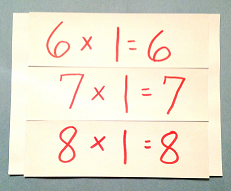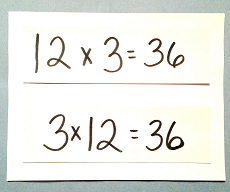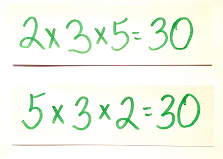# Multiplication Properties Lesson for Kids

Instructor: Audrey Akins

Audrey has more than a decade of experience teaching elementary. She has a bachelor's in journalism and a master's in education.

In this lesson, we'll discuss multiplications properties. You'll learn about the four properties of multiplication and how they work, then you can test your knowledge with a quick quiz.

## Multiplication Properties

Learning how to multiply doesn't have to be hard. There are four multiplications properties that can help make the process easier: the identity, commutative, associative and distributive properties. They provide rules for quickly solving multiplication problems according to properties that always apply to certain equations. Let's explore these multiplications properties below.

## Identity PropertyThe rule for the identity property is that a number multiplied by 1 will always yield that number as the answer, or product. Think of it like this: When multiplied by 1, a number does not change its identity--it always stays the same.

Imagine a superhero named 7 Man, dressed up in a mask and cape. When 1 comes at him to try and change him, 7 Man goes unaltered. He comes out of the multiplication problem just the same, wearing his mask and cape. So, 7 x 1 = 7.

## Commutative PropertyCommutative property states that two numbers multiplied together will always produce the same answer no matter which number is first or second in the problem. This means 12 x 3 = 36 and 3 x 12 = 36. The same for 7 x 9 = 63 and 9 x 7 = 63. The rule works for any two numbers being multiplied together.

## Associative PropertySimilar to the commutative property is the associative property. The rule for the associative property is that when three numbers are multiplied together, the product will always be the same no matter which number is first, second, or third. For example, 2 x 3 x 5 = 30 and 5 x 3 x 2 = 30. Also, 3 x 5 x 2 = 30.

## Distributive Property

Distributive property says that when you have an equation such as 2 x (3 + 4), the answer will be the same, whether or not you choose to solve it the way it's written or to distribute before solving: (2 x 3) + (2 x 4). We'll solve the problems both ways to clarify.

To unlock this lesson you must be a Study.com Member.

### Register to view this lesson

Are you a student or a teacher?

#### See for yourself why 30 million people use Study.com

##### Become a Study.com member and start learning now.
Back
What teachers are saying about Study.com

### Earning College Credit

Did you know… We have over 200 college courses that prepare you to earn credit by exam that is accepted by over 1,500 colleges and universities. You can test out of the first two years of college and save thousands off your degree. Anyone can earn credit-by-exam regardless of age or education level.